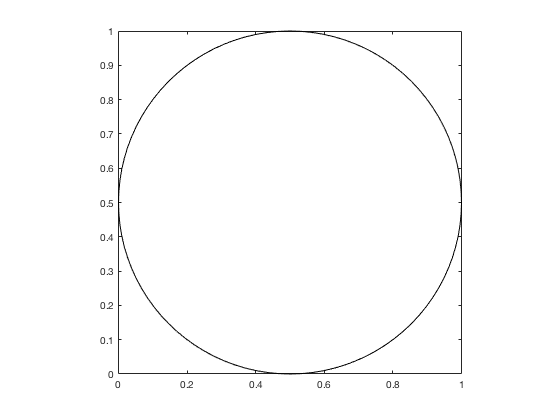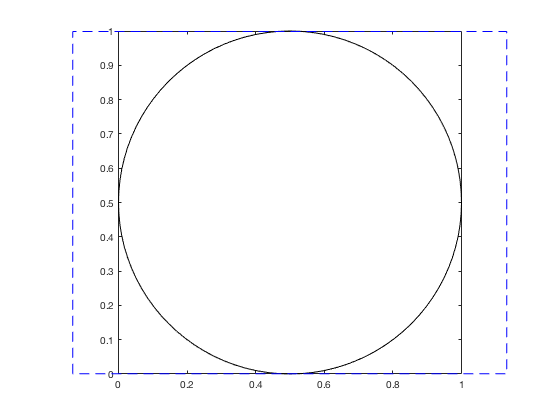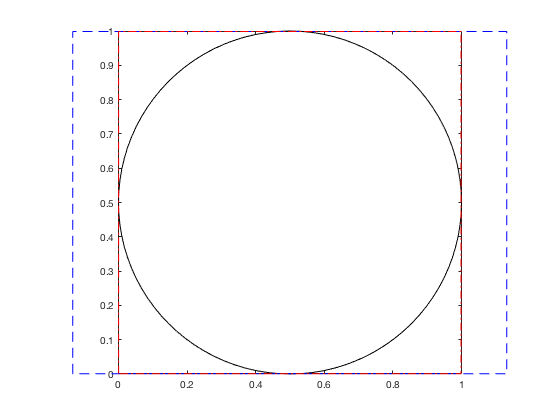## plotboxpos

버전 1.4.0.1 (41.7 KB) 작성자:
Returns the position of a plotted axis region

다운로드 수: 5.1K

업데이트 날짜: 2021/9/23

GitHub에서 호스트

# plotboxpos.m: Plot box (visible) position

This repository includes the code for the `plotboxpos.m` Matlab function.

This function returns the position of the plotted region of an axis, which may differ from the actual axis position, depending on the axis limits, data aspect ratio, and plot box aspect ratio. The position is returned in the same units as the those used to define the axis itself. This function can only be used for a 2D plot.

## Contents

• Getting started
• Syntax
• Examples
• Contributions

## Getting started

Prerequisites

This function requires Matlab R14 or later.

This code can be downloaded from Github or the MatlabCentral File Exchange. The File Exchange entry is updated daily from the GitHub repository.

Matlab Search Path

The following folders need to be added to your Matlab Search path (via `addpath`, `pathtool`, etc.):

`plotboxpos-pkg/plotboxpos`

## Syntax

`pos = plotboxpos(h)` returns the 1 x 4 position vector `pos` for the axis with handle `h`. The units of `pos` will match those of the axis' parent object (typically the figure).

## Examples

We start by plotting a circle, changing the axis aspect ratio to be 1:1 and the axis limits to be tight to the data.

```h.ax(1) = axes;
h.ex = rectangle('position', [0 0 1 1], 'curvature', [1 1]);
axis tight equal;
box on;```The axis `'Position'` property still corresponds to the full potential axis space, discounting the axis modifications we just made.

```pos1 = get(h.ax(1), 'position');
annotation('rectangle', pos1, 'edgecolor', 'b', 'linestyle', '--');```The `plotboxpos` function returns the position the axis is actually using with its current axis ratio settings;

```pos2 = plotboxpos(h.ax(1));
annotation('rectangle', pos2, 'edgecolor', 'r', 'linestyle', '-.');```## Contributions

Community contributions to this package are welcome!

To report bugs, please submit an issue on GitHub and include:

• your version of Matlab and all relevant toolboxes (type `ver` at the Matlab command line to get this info)
• code/data to reproduce the error or buggy behavior, and the full text of any error messages received

Please also feel free to submit enhancement requests, or to send pull requests (via GitHub) for bug fixes or new features.

I do monitor the MatlabCentral FileExchange entry for any issues raised in the comments, but would prefer to track issues on GitHub.

Published with MATLAB R2016b

### 인용 양식

Kelly Kearney (2023). plotboxpos (https://github.com/kakearney/plotboxpos-pkg), GitHub. 검색됨 .

개발 환경: R14SP2
모든 릴리스와 호환
##### 플랫폼 호환성
Windows macOS Linux
##### 카테고리
Help CenterMATLAB Answers에서 Aspect Ratios and Layout에 대해 자세히 알아보기

### Community Treasure Hunt

Find the treasures in MATLAB Central and discover how the community can help you!

Start Hunting!

#### plotboxpos

GitHub 디폴트 브랜치를 사용하는 버전은 다운로드할 수 없음

버전 게시됨 릴리스 정보
1.4.0.1

1.4.0.0

Updated description to match the one on GitHub.

1.3.0.0

- verified for R2014b graphics

1.2.0.0

Now defaults to current axis if no input provided. Also updated example image to a simpler one.

1.1.0.0

Small rewrite to remove dependency on external functions

1.0.0.0

Fixed incorrect behavior when aspect ratios set to 'auto', and some bugs regarding plot box aspect ratio.

이 GitHub 애드온의 문제를 보거나 보고하려면 GitHub 리포지토리로 가십시오.
이 GitHub 애드온의 문제를 보거나 보고하려면 GitHub 리포지토리로 가십시오.